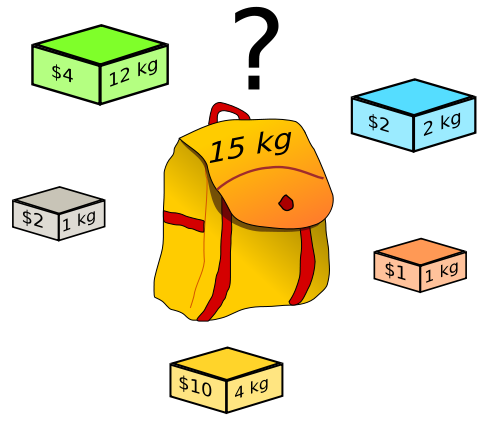# 0-1 Knapsack Problem in C Using Dynamic Programming

Here you will learn about 0-1 knapsack problem in C.

If you are interested in learning more about dynamic programming techniques, AlgoMonster’s Dynamic Programming Introduction and Dynamic Programming Patterns.

We are given n items with some weights and corresponding values and a knapsack of capacity W. The items should be placed in the knapsack in such a way that the total value is maximum and total weight should be less than knapsack capacity.

In this problem 0-1 means that we can’t put the items in fraction. Either put the complete item or ignore it. Below is the solution for this problem in C using dynamic programming.

##Program for Knapsack Problem in C Using Dynamic Programming

Output

Enter number of items:3
Enter value and weight of items:
100 20
50 10
150 30
Enter size of knapsack:50
250

You can watch below video to learn knapsack problem easily.

### 11 thoughts on “0-1 Knapsack Problem in C Using Dynamic Programming”

1.thanks , really a grate article.

2.effective programing

3.For learning programming, Your website is the best Neeraz.

4.What does the val array contain?Thanks in advance

5.this program is not defind in the java using c

6.int max(int a, int b) { return (a > b)? a : b; }

Require of this line ???

7.Hey hai , this chandru..
I need an explanation on the condition

1. Else if(wt[i-1]<=w) // this means that weight at index [i-1] is compared with index w
where weight values are [20,10,30 ] and w[0 to n]..

so when comparing wt[i-1]<=w which will never be a true.. If i am right!!!

1.w is not from 0 to n. w is from 0 to W. here in this case w[0 to 50].

8.Thanks for this great tutorial. I have tried to change the code, because I need to show the selected Items, but it doesn’t work.

Maybe Someone have a solution that show the chosen items ?

Thanks for help

9.Anushree Chatterjee

What if we need to solve it using backtracking approach??

10.Thank you a lot for the program.
You offer me 2 bonus points on my final exam.
Love on you <3